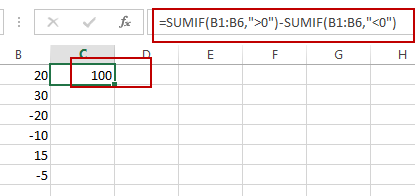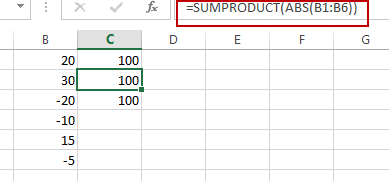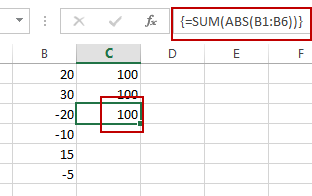# Sum the Absolute Values in Excel

This post will guide you how to sum the absolute values in a range of cells in Excel. How do I sum the absolute values with a formula in Excel 2013/2016.

## Sum the Absolute Values

Assuming that you have a list of data in range B1:B6 that contain negative numbers and positive numbers, and you want to sum all absolute values in these range of cells, how to achieve it.

You can use a formula based on the SUMIF function to achieve the result. Like this:

`=SUMIF(B1:B6,”>0”)-SUMIF(B1:B6,”<0”)`

Type this formula into a blank cell and then press Enter key in your keyboard.The sum of the absolute values have been calculated.

You can also use another formula based on the SUMPRODUCT function and the ABS function to achieve the same result. Like this:

`=SUMPRODUCT(ABS(B1:B6))`

Type it into the formula box of a blank cell, then press Enter key.You can also use an Excel Array formula based on the SUM function and the ABS function to sum the absolute values in range B1:B6. Like this:

`=SUM(ABS(B1:B6))`

You need to type this formula into a cell, and then press Ctrl + Shift + Enter keys in your keyboard.### Related Functions

• Excel SUM function
The Excel SUM function will adds all numbers in a range of cells and returns the sum of these values. You can add individual values, cell references or ranges in excel.The syntax of the SUM function is as below:= SUM(number1,[number2],…)…
• Excel SUMPRODUCT function
The Excel SUMPRODUCT function multiplies corresponding components in the given one or more arrays or ranges, and returns the sum of those products.The syntax of the SUMPRODUCT function is as below:= SUMPRODUCT (array1,[array2],…)…
• Excel SUMIF Function
The Excel SUMIF function sum the numbers in the range of cells that meet a single criteria that you specify. The syntax of the SUMIF function is as below:=SUMIF (range, criteria, [sum_range])…
• Excel ABS Function
The Excel ABS function returns the absolute value of a number.The ABS function is a build-in function in Microsoft Excel and it is categorized as a Math and Trigonometry Function.The syntax of the ABS function is as below:=ABS (number)…